## Introduction and background

In this multi-part series of articles, I will document my efforts in writing a high school Philosophy paper using ChatGPT. The paper shall answer in writing a question I was asked at an impromptu oral assessment in Philosophy class in my 3rd year of high school.

Let me take a step back to give you some context. I was enrolled in Classical High School in Rome, Italy. This type of school has a strong 5-year emphasis on History and Philosophy, as well as Latin, Greek, and Italian literature and grammar, with a bit of Science and Math. Students are typically evaluated on weekly written assignments, a minimum of 3 scheduled written tests each semester, and a minimum of 2 oral assessments per semester. During the latter ones, the teacher would (usually) pick two names at random from the attendance list once a week and gauge their knowledge on both the weekly lesson, in detail, but also on anything covered in the year up to that point; this could last between 30-60 minutes, and would happen in front of an audience of (often roaring) classmates. Seriously: brutal!

Only occasionally were students allowed to give a presentation on a prepared topic or volunteer for a full oral evaluation. This was the case for me during a block on Plato. And so it was that the teacher, the famously feared Professoressa Carbone (who taught both History and Philosophy), probed into my soul with her terrifying eyes and asked with a sinister smile, “Tell me, Niccoli, what would Plato have thought about the Hundred Years’ War?

## My first prompt

When I heard about OpenAI ChatGPT being available, it seemed very fit to try this question in my first investigation. So I went ahead and asked the question “What would Plato have thought about the Hundred Years’ War?“, with only the minor modification to add citations and break down the response in steps.

## GPT-3.5 result

Below is the result from the default GPT-3.5 model:

## Time investment and return

• Time investment: the whole investigatyion took about 30 minutes, including a very light read on prompts from a reputalbe source, and reading the answer (becaue the citations really did not come as full references, there was really nothing else to do other than that).
• Return: was this worth 30 minutes of my time? The answer is, as often, it depends! On the one hand, this is a really poor answer in my view (see next section for a more detailed evaluation). So, as an essay (l imagine myself as a student looking for a quick free lunch), this would not do it; not even close. For me… but then again, even at 13 in my first year of high school I would’ve known this was not going to cut it… or in Junior high, for what matters. On the other hand, I was deliberate in providing a very simple, not engineered or iteratively refined prompt. As such, the response will come useful as a benchamrk against wich I will compare other efforts (for example using a better model, GPT4, and improving the prompt).

## Evaluation

Is the answer grammatically correct? yes; is it written in a uniform style, appropriate for a school essay? yes; Does it contain any hallucination? I would say no, everything sounds reasonable. However I must admit that without reference to specific passages to Plato’s work, it is hard to say for sure. From what I remember of Plato’s Philosophy it’s reasonable, and that’s all.

On the other hand, had I been handed in this as a teacher, I would not be impressed. IF this were the output of an in-class exam, I probably would have marked it as a D- (a D for the very mediocre text and a minus for the ridiculousness of the citations). Had it instead been handed for an at home written assignement (in which cse the student would have had access to their notes and books) this would definitely be certainly worth an F (Fail). Now I sound like Professoressa Carbone.

You may reasonably wonder at the end of this article: how did I fare in that evaluation in 1986? Fair question. And I am not ashamed to confess it was a disaster. At 15 I was still very immature, intelligent but unengaged in school work, and also unable to do a real analysis. By that I mean interpret information rather than just accumulate facts and connect them. I honestly do not recall the mark I got (we had different levels of Failure that went progressively deeper, not too dissimilarly from the Circles in Dante’s Inferno) but I rememberr that an F definitely it was.

P.S. The model did a perfectly fine job in proofreading my first draft for this post. THat was definitely worth a lot, given I type very slowly, and I make lots of typos.

## Playtime: explorations with OpenAI’s GPT-4, introduction

### Featured

I will be honest. I have been very busy and unable to write much. Specifically on the latest advances in large language models and their applications, I had forced myself to stay on the fence. Yes, read about it, stay on top of the developments, but leave the experimenting to others, for the time being. Then two things changed my mind: first, I started working through David Mertz’ book Regular Expression Puzzles and AI Coding Assistants (very superficially, admittedly, as regular expressions are not my forte, but the book is awesome); second, I chanced on the article Why everyone should try GPT-4, even the CEO by Cassie Kozyrkov.

Here are the things I am actively playing with, right now. Soon I will start documenting these explorations with a new series of short blog posts:

• Writing an essay in philosophy based on a specific, complex prompt
• Act as baking assistant
• Write an imaginary dialogue between specific historic and fictional characters
• Demonstrate geometry theorem
• Perform arithmetic operations
• Act as song translation assistant
• Write poem with specific metric, and music in a specific composer’s style
• Act as coding assistant
• Solve puzzles
• Play a live tic tac toe and/or chess game
• Detect logical fallacies
• Help with complex numerical estimations given a set of rules

## 10-year anniversary

### Featured

July 15th, 2022

I started writing this blog 10 years ago and it is to date one of the things I am the most proud of.

This is a big milestone for me, so I would like to begin with thanking all the people that encouraged me at the beginning, and in particular, for their valiant support and feedback: Matt Hall, Evan Bianco, Oliver Kuhn, Steve Lynch, and last but not least my life partner Rebecca.

A lot of the work I did in the first couple of years was on furthering my understanding, and sharing that with others, of the use of colours in scientific visualization, and how to use better colormaps, for example Perceptual rainbow palette, part a and part b.

I am grateful I achieved those knowledge-sharing goals:

• The material is referenced in the matplotlib documentation
• The blog has often been used as reference in talks and other publications on colormaps, beginning with this classic matplotlib talk given by Kristen Thyng at scipy 2014
• I was thrilled to have received positive feedback on my work by Bernice Rogovitz, someone I hold in very high esteem
• Some of that work on the blog resulted in being invited by Matt Hall to write a tutorial for The Leading Edge (SEG). The tutorial came with a Jupyter notebook demonstrating how to evaluate default colour maps and favour more perceptual alternatives
• I am particularly proud to see that the article is still ranking in the top 20 most downloaded papers from The Leading Edge (between 2010-2020)
• Additionally, the two blog post are to date top results for the #google search “perceptual rainbow” and “perceptual palette images

Ultimately, I am very happy to have created a space for sharing and exchanging ideas freely.

So, to celebrate these 10 years of MyCarta, I treated it to a new domain, mycartablog.com (but the old domain, and links still work) and a brand new look (it took me a while to get there but I like it a lot) with a theme that should now be responsive for all devices (welcome to the new era Matteo!).

I will also soon publish a short series of short but sweet new posts on colormaps and visualization (and republish on linkedin).

Thank you all for sharing this journey!

### Featured

October 22, 2020

In my last post I touched on the topic of continuously improving your geo-computing projects (also take a look at my chapter from the upcoming Software Underground book, 52 things you should know about geocomputing).

However, one aspect that I intentionally left out in was that of coding skills as I was planning to get back to it with a dedicated post, which you are reading just now.

#### 2018 vs 2020 comparison of flag percentage calculation

In the Jupyter notebook I compare the results of seismic inversion from two methods (with or without inversion-tailored noise attenuation) using a custom function to flag poor prediction of the target well log using median/median absolute deviation as a statistic for the error; the results are shown below.

One may just do this visual comparison, but I also included calculations to count the number and percentage of samples that have been flagged for each case. Below is a cell of code from the Jupyter notebook (let’s call it 2020 code) that does just that .

``zone_errors_a['flagged samples']=result_a.groupby('zone', sort=False).flag.sum().valueszone_errors_b['flagged samples']=result_b.groupby('zone', sort=False).flag.sum().valuesdef calc_proportion(dtf):    """    function to calculate proportion of flagged samples    """    x=dtf.flag    return round(100 * x.sum()/len(x), 1)zone_errors_a['proportion (%)']=result_a.groupby('zone',sort=False).apply(calc_proportion).valueszone_errors_b['proportion (%)']=result_b.groupby('zone',sort=False).apply(calc_proportion).values``

I am a lot happier with this code than with the original code (circa 2018), which is in the cell below.

``````zones_a=list(result_a['zone'].unique())
zones_b=list(result_b['zone'].unique())

zone_errors_a['flagged samples']=[result_a.loc[result_a.zone==z,'flag'].sum() for z in zones_a]
zone_errors_b['flagged samples']=[result_b.loc[result_b.zone==z,'flag'].sum() for z in zones_b]

zone_errors_a['proportion (%)']=[round(result_a.loc[result_a.zone==z,  'flag'].sum()/len(result_a.loc[result_a.zone==z,'flag'])*100,1) for z in zones_a]

zone_errors_b['proportion (%)']=[round(result_b.loc[result_b.zone==z,  'flag'].sum()/len(result_b.loc[result_b.zone==z,'flag'])*100,1) for z in zones_b]                                    ``````

The major differences in the older code are:

• I was using `unique` instead of Pandas’ `groupby`
• I was using list comprehensions to work through the DataFrame, instead of Pandas’ `apply` and a custom function to calculate the percentages on the entire DataFrame at once.

I find the 2020 code much more tidy and easier to read.

#### Enters Pandas for everyone

The above changes happened in but a few hours over two evenings, after having worked through chapters 9 and 10 of Pandas for Everyone by Daniel Chen, a very accessible read for all aspiring data scientists, which I highly recommend (also, watch Daniel’s fully-packed 2019 Pycon tutorial).

And before you ask: no, you do not get the Agile Scientific sticker with the book, I am sorry.

🙂

#### Comparison of 2016 vs 2020 code snippets from the 2016 SEG Machine Learning contest

A second example is of code used to calculate the first and second derivatives for all geophysical logs from the wells in the 2016 SEG Machine Learning contest.

The two cells of code below do exactly the same thing: loop through the wells and for each one in turn loop through the logs, calculate the derivatives, add them to a temporary Pandas DataFrame, then concatenate into a single output DataFrame. In this case, the only difference is the moving away from `unique` to `groupby`.

I use the `%%timeit` cell magic to compare the runtimes for the two cells.

###### 2016 code
``````%%timeit
# for training data
# calculate all 1st and 2nd derivative for all logs, for all wells
train_deriv_df = pd.DataFrame()             # final dataframe

for well in train_data['Well Name'].unique():        # for each well
new_df = pd.DataFrame() # make a new temporary dataframe

for log in ['GR', 'ILD_log10', 'DeltaPHI', 'PHIND' ,'PE']: # for each log
# calculate and write to temporary dataframe
new_df[str(log) + '_d1'] = np.array(np.gradient(train_feat_df[log][train_feat_df['Well Name'] == well]))

# append all rows of temporary dataframe to final dataframe
train_deriv_df = pd.concat([train_deriv_df, new_df])

86 ms ± 1.47 ms per loop (mean ± std. dev. of 7 runs, 10 loops each)``````
###### 2020 code
``````%%timeit
# for training data
# calculate all 1st and 2nd derivative for all logs, for all wells
train_deriv_df = pd.DataFrame() # final dataframe

for _, data in train_feat_df.groupby('Well Name'): # for each well
new_df = pd.DataFrame()                        # make a new temporary dataframe

for log in ['GR', 'ILD_log10', 'DeltaPHI', 'PHIND' ,'PE']: # for each log
# calculate and write to temporary dataframe

# append all rows of temporary dataframe to final dataframe
train_deriv_df = pd.concat([train_deriv_df, new_df])

52.3 ms ± 353 µs per loop (mean ± std. dev. of 7 runs, 10 loops each)``````

We go down to 52.3 ms from 86 ms, which is a modest improvement, but certainly the code is more compact and a whole lot lighter to read (i.e. more pythonic, or pandaish if you prefer): I am happy!

As an aside, if you want to know more about timing code execution, see section 1.07 from Jake VanderPlas’ outstanding Python Data Science Handbook, which I also cannot recommend enough (and do yourself a favor: watch his series Reproducible Data Analysis in Jupyter).

By the way, below I show the notebook code comparison generated using the nbdiff-web option from the awesome `nbdime` library, a recent discovery.

## Be a geoscience and data science detective

### Featured

September 16, 2020

#### Introduction

These days everyone talks about data science. But here’s a question: if you are a geoscientist, and like me you have some interest in data science (that is, doing more quantitative and statistical analyses with data), why choose between the two? Do both… always! Your domain knowledge is an indispensable condition, and so is an attitude of active curiosity (for me, an even more important condition). So, my advice for aspiring geoscientists and data scientists is: “yes, do some course work, read some articles, maybe a book, get the basics of Python or R, if you do not know a programming language” but then jump right ahead into doing! But – please! – skip the Titanic, Iris, Cars datasets, or any other data from your MOOC or that many have already looked at! Get some data that is interesting to you as geoscientist, or put it together yourself.

#### First example: evaluate the quality of seismic inversion from a published article

In this first example, the one presented at Transform 2020, I wanted to evaluate the quality of seismic inversion from a published article in the November 2009 CSEG Recorder Inversion Driven Processing. In the article, the evaluation was done by the authors at a blind well location, but only qualitatively, as illustrated in Figure 5, shown below for reference. In the top panel (a) the evaluation is for the inversion without additional processing (SPNA, Signal Protected Noise Attenuation); in the bottom panel (b) the evaluation is for the inversion with SPNA. On the right side of each panel the inverted seismic trace is plotted against the upscaled impedance well log (calculated by multiplying the well density log and the well velocity log from compressional sonic); on the right, the upscaled impedance log is inserted in a seismic impedance section as a colored trace (at the location of the well) using the same color scale and range used for the impedance section.Figure 5 caption: Acoustic impedance results at the blind well for data without (a) and with (b) SPNA. The figure shows a 200 ms window of inverted seismic data with well B, the blind well, in the middle on the left, along with acoustic impedance curves for the well (red) and inverted seismic (blue) on the right. The data with SPNA shows a better fit to the well, particularly over the low frequencies.

What the authors reported in the figure caption is the extent to which the evaluation was discussed in the paper; unfortunately it is not backed up in any quantitative way, for example comparing a score, such as R^2, for the two methods. Please notice that I am not picking on this paper in particular, which in fact I rather quite liked, but I am critical of the lack of supporting statistics, and wanted to supplement the paper with my own.

In order to do that, I hand-digitized from the figure above the logs and inversion traces , then interpolated to regularly-sampled time intervals (by the way: if you are interested in a free tool to  digitize plots, check  use WebPlotDigitizer).

My plan was to split my evaluation in an upper and lower zone, but rather than using the seismically-picked horizon, I decided to add a fake top at 1.715 seconds, where I see a sharp increase in impedance in Figure 5. This was an arbitrary choice on my part of a more geological horizon, separating the yellow-red band from the green blue band in the impedance sections. The figure below shows all the data in a Matplotlib figure:The first thing I did then, was to look at the Root Mean Square Error in the upper and lower zone obtained using the fake top. They are summarized in the table below: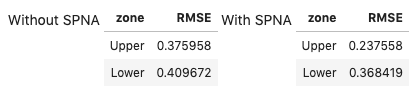Based on the RMSE , it looks like case b, the inversion with Signal Protected Noise Attenuated applied on the data, is a better result for the `Upper` zone, but not for the `Lower` one. This result is in agreement with my visual comparison of the two methods.

But lets’ dig a bit deeper. After looking at RMSE, I used the updated version of the `error_flag` function, which I first wrote at the 2018 Geophysics Sprint, listed below:

```def error_flag(pred, actual, stat, dev = 1.0, method = 1):
"""Calculate the difference between a predicted and an actual curve
and return a curve flagging large differences based on a user-defined distance
(in deviation units) from either the mean difference or the median difference

Matteo Niccoli, October 2018. Updated in May 2020.

Parameters:
predicted : array
predicted log array
actual : array
original log array
stat : {‘mean’, ‘median’}
The statistics to use. The following options are available:
- mean: uses numpy.mean for the statistic,
and np.std for dev calculation
- median: uses numpy.median for the statistic,
and scipy.stats.median_absolute_deviation (MAD) for dev calculation
dev : float, optional
the standard deviations to use. The default is 1.0
method : int {1, 2, 3}, optional
The error method to use. The following options are available
(default is 1):
1: difference between curves larger than mean difference plus dev
2: curve slopes have opposite sign (after a 3-sample window smoothing)
3: curve slopes of opposite sign OR difference larger than mean plus dev
Returns:
flag : array
The error flag array
"""

flag = np.zeros(len(pred))
err = np.abs(pred-actual)

if stat == 'mean':
err_stat = np.mean(err)
err_dev = np.std(err)
elif stat == 'median':
err_stat = np.median(err)
err_dev = sp.stats.median_absolute_deviation(err)

pred_sm = pd.Series(np.convolve(pred, np.ones(3), 'same'))
actual_sm = pd.Series(np.convolve(actual, np.ones(3), 'same'))
ss = np.sign(pred_sm.diff().fillna(pred_sm))
ls = np.sign(actual_sm.diff().fillna(actual_sm))

if method == 1:
flag[np.where(err>(err_stat + (dev*err_dev)))] = 1
elif method == 2:
flag[np.where((ss + ls)==0 )]= 1
elif method == 3:
flag[np.where(np.logical_or(err>(err_stat + (dev*err_dev)), (ss+ls)==0 ))]= 1
return flag```

I believe this new version is greatly improved because:

• Users now can choose between mean/standard deviation and median/median absolute deviation as a statistic for the error. The latter is more robust in the presence of outliers
• I added a convolutional smoother prior to the slope calculation, so as to make it less sensitive to noisy samples
• I expanded and improved the doctstring

The figure below uses the flag returned by the function to highlight areas of poorer inversion results, which I assigned based on passed to the function very restrictive parameters:

• using median and a median absolute deviation of 0.5 to trigger flag
• combining the above with checking for the slope signI also wrote short routines to count the number and percentage of samples that have been flagged, for each result, which are summarized in the table below: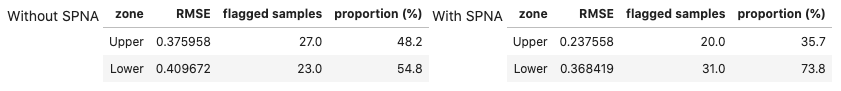The error flag method is in agreement with the RMS result: case b, the inversion on Signal Protected Noise Attenuated data is a better result for the `Upper` zone, but for the `Lower` zone the inversion without SPNA is the better one. Very cool!

But I was not satisfied yet. I was inspired to probe even deeper after a number of conversations with my friend Thomas Speidel, and reading the chapter on Estimation from Computational and Inferential Thinking (UC Berkeley). Specifically, I was left with the question in mind: “Can we be confident about those two is better in the same way?

This question can be answered with a bootstrapped Confidence Interval for the proportions of flagged samples, which I do below using the code in the book, with some modifications and some other tools from the datascience library. The results are shown below. The two plots, one for the `Upper` and one for the `Lower` zone, show the distribution of bootstrap flagged proportions for the two inversion results, with SPNA in yellow, and without SPNA in blue, respectively, and the Confidence Intervals in cyan and brown, respectively (the CI upper and lower bounds are also added to the table, for convenience).

By comparing the amount (or paucity) of overlap between the distributions (and between the confidence intervals) in the two plots, I believe I can be more confident in the conclusion drawn for the Lower zone, which is that the inversion on data without SPNA is better (less proportion of flagged errors), as there is far less overlap.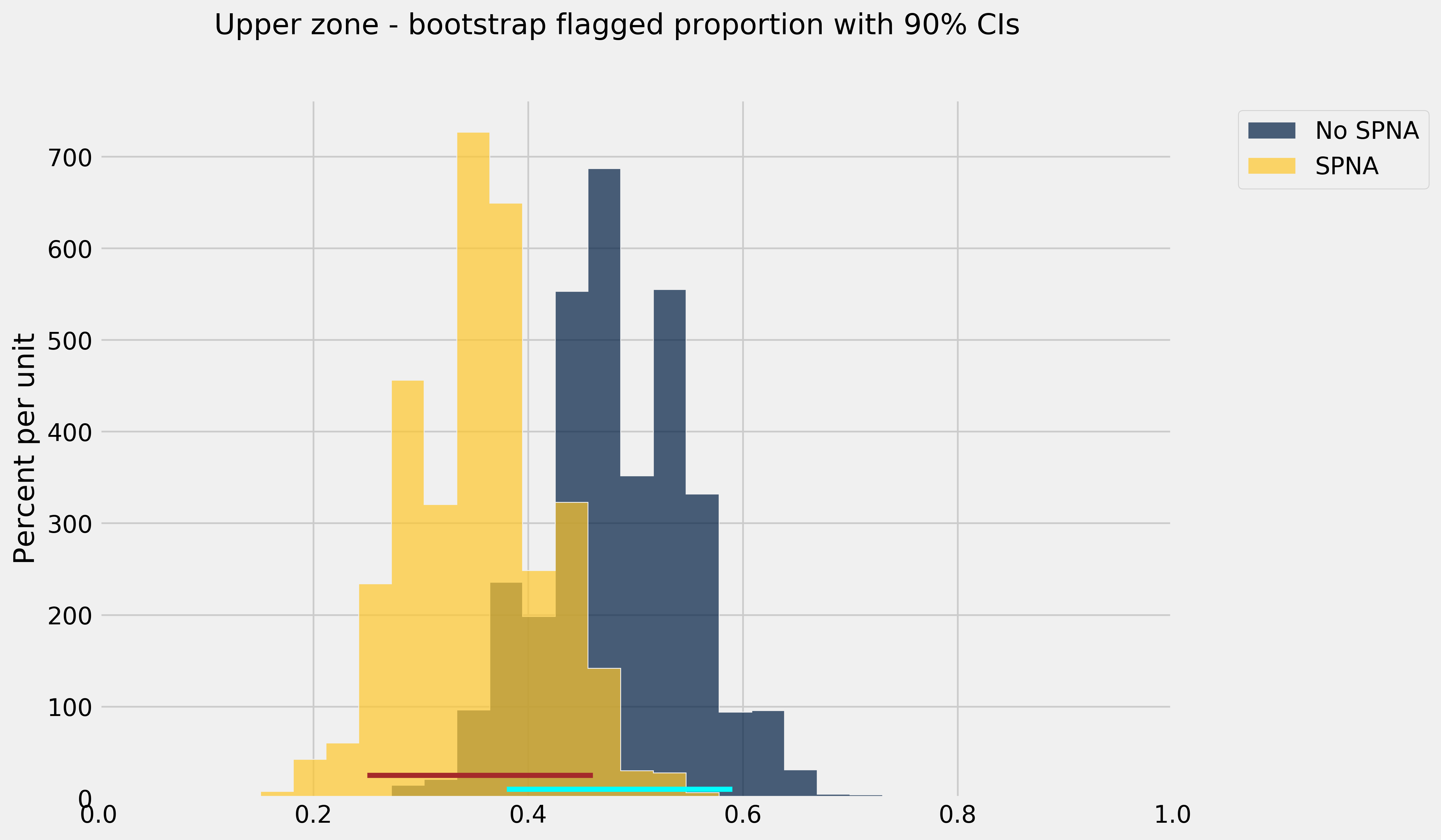I am very pleased with these results. Of course, there are some caveats to keep in mind, mainly that:
• I may have introduced small, but perhaps significant errors with hand digitizing
• I chose a statistical measure (the median and median absolute deviation) over a number of possible ones
• I chose an arbitrary geologic reference horizon without a better understanding of the reservoir and the data, just the knowledge from the figure in the paper

However, I am satisfied with this work, because it points to a methodology that I can use in the future. And I am happy to share it! The Jupyter notebook is available on GitHub.

#### Second example: evaluate regression results from a published article

My second, more recent example, is shorter but no less interesting, in my opinion. It is a case study correlating inversion data to petrophysical estimates of porosity-height in the Doig and Montney Formations in Western Canada (from the paper Tight gas geophysics: AVO inversion for reservoir characterization, Close et al. CSEG Recorder, May 2010, an article which I enjoyed very much reading).

The authors indicated that Vp/Vs and/or Poisson’s ratio maps from seismic inversion are good indicators of porosity in the Lower Doig and Upper Montney reservoirs in the wells used in their study, so it was reasonable to try to predict Phi-H from Vp/Vs via regression. The figure below, from the article, shows one such Vp/Vs ratio map and the Vp/Vs vs. Phi-H cross-plot for 8 wells.Figure 7 caption: Figure 7. a) Map of median Vp/Vs ratio value and porosity-height from 8 wells through the Lower Doig and Upper Montney. The red arrows highlight wells with very small porosity-height values and correspond in general to areas of higher Vp/Vs ratio. The blue arrow highlights a well at the edge of the seismic data where the inversion is adversely affected by decreased fold. The yellow line is the approximate location of a horizontal well where micro-seismic data were recorded during stimulation. b) Cross-plot of porosity-height values as shown in (a) against Vp/Vs extracted from the map. The correlation co-efficient of all data points (blue line) of 0.7 is improved to 0.9 (red line) by removing the data point marked by the blue arrow which again corresponds to the well near the edge of the survey (a).

They also show in the figure that by removing one of the data points, corresponding to a well near the edge of the survey (where the seismic inversion result is presumably not as reliable, due to lower offset and azimuth coverage), the correlation co-efficient is improved from 0.7 to 0.9 (red line).

So, the first thing I set out to do was to reproduce the crossplot.  I again hand-digitized the porosity-height and Vp/Vs pairs in the cross-plot using again WebPlotDigitizer. However, switched around the axes, which seems more natural to me since the objectives of regression efforts would be to predict as the dependent variable Phi-h, at the location of yet to drill wells, given Vp/Vs from seismic inversion. I also labelled the wells using their row index, after having loaded them in a Pandas DataFrame. And I used Ordinary Least Square Regression from the `statsmodels` library twice: once with all data points, the second time after removal of the well labelled as 5 in my plot above.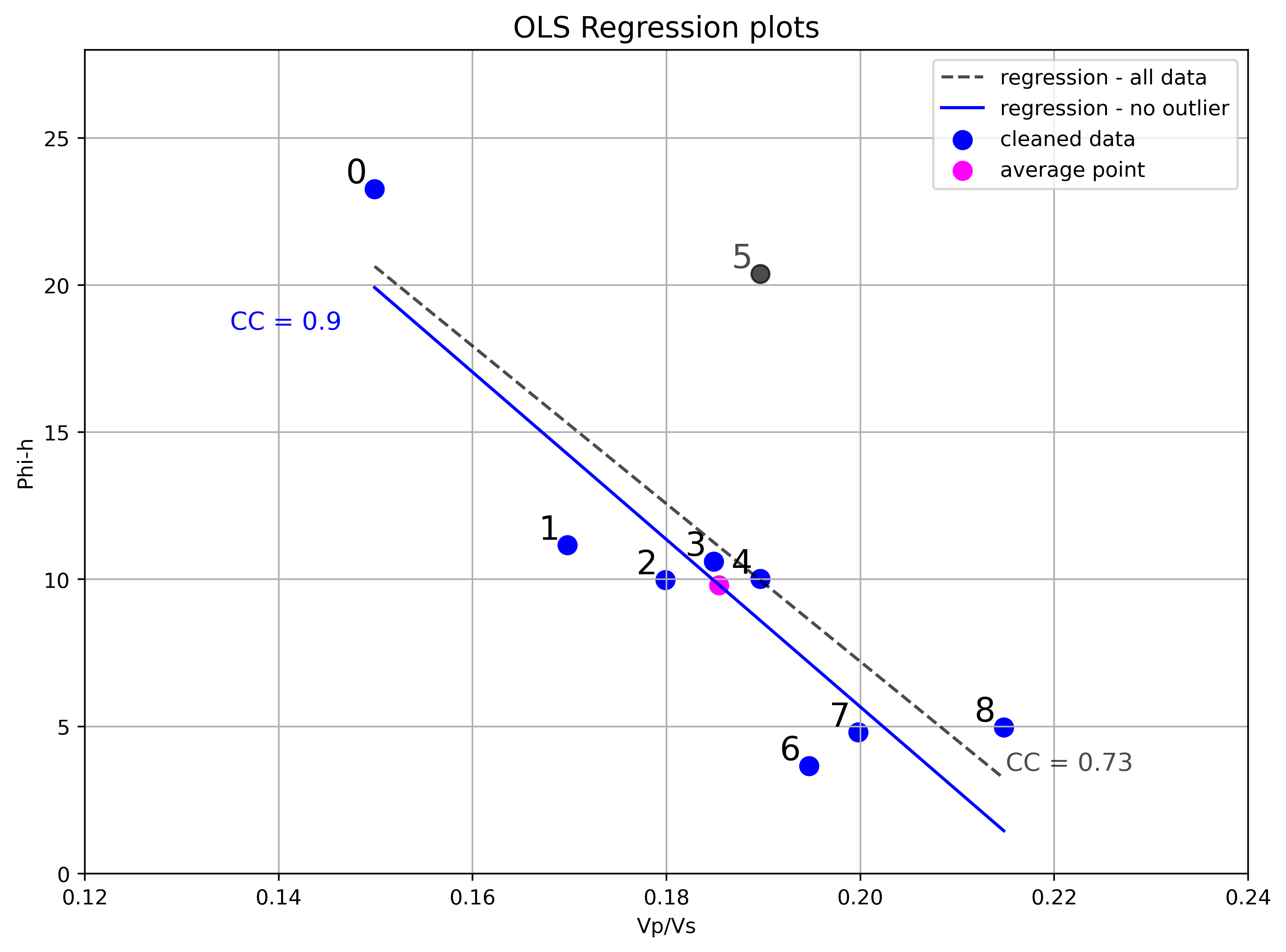So, I am able to reproduced the analysis from the figure. I think removing an outlier with insight from domain knowledge (the observation that poorer inversion result at this location is reasonable for the deviation from trend) is a legitimate choice. However, I would like to dig a bit deeper, to back up the decision with other analyses and tests, and to show how one might do it with their own data.

The first thing to look at is an Influence plot, which is a plot of the residuals, scaled by their standard deviation, against the leverage, for each observation. Influence plots are useful to distinguish between high leverage observations from outliers and are one of `statsmodel` ‘s standard Regression plots, so we get the next figure almost for free, with minor modifications to the default example). Here it is below, together with the OLS regression result, with all data points.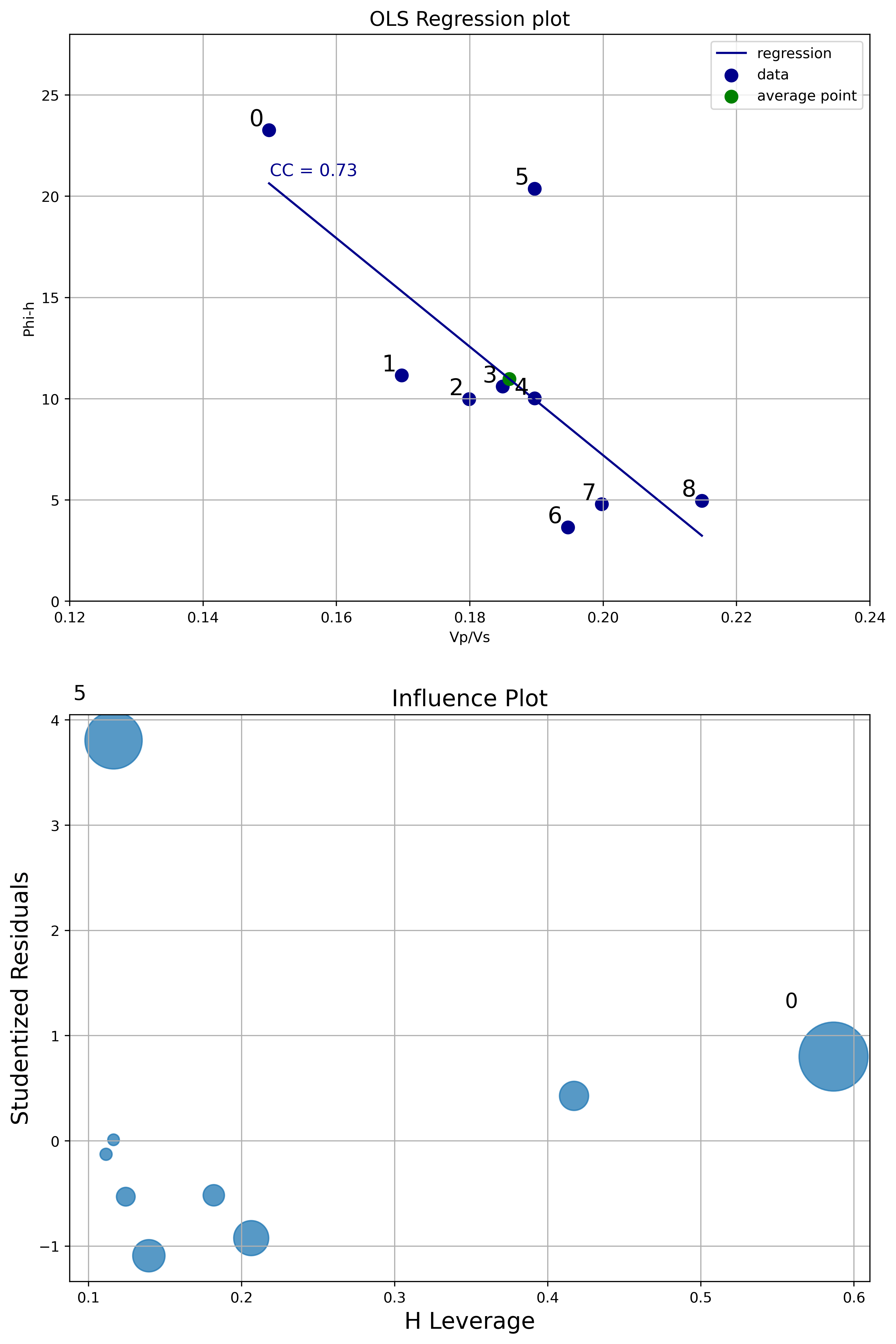From the Influence plot it is very obvious that the point labelled as zero has high leverage (but not high normalized residual). This is not a concern because points with high leverage are important but do not alter much the regression model. On the other hand, the point labelled as 5 has very high normalized residual. This point is an outlier and it will influence the regression line, reducing the R^2 and correlation coefficient. This analysis is a robust way to legitimize removing that data point.

Next I run some inferential tests. As I’ve written in an earlier notebook on Data loading, visualization, significance testing, I find the critical r very useful in the context of bivariate analysis. The critical r is the value of the correlation coefficient at which you can rule out chance as an explanation for the relationship between variables observed in the sample, and I look at it in combination with the confidence interval of the correlation coefficient.

The two plots below display, in dark blue and light blue respectively, the upper and lower confidence interval bounds, as the correlation coefficient r varies between 0 and 1 (x axis). These two bounds will change with different number of wells (they will get closer with more wells, and farther apart with less wells). The lower bound intersects the x axis (y=0) axis at a value equal to the critical r (white dot). The green dots highlight the actual confidence interval for a specific correlation coefficient chosen, in this case 0.73 with 9 wells, and 0.9 with 8 wells.

By the way: these plots are screen captures from the interactive tool I built taking advantage of the Jupyter interactive functionality (`ipywidgets`). You can try the tool by running the Jupyter notebook.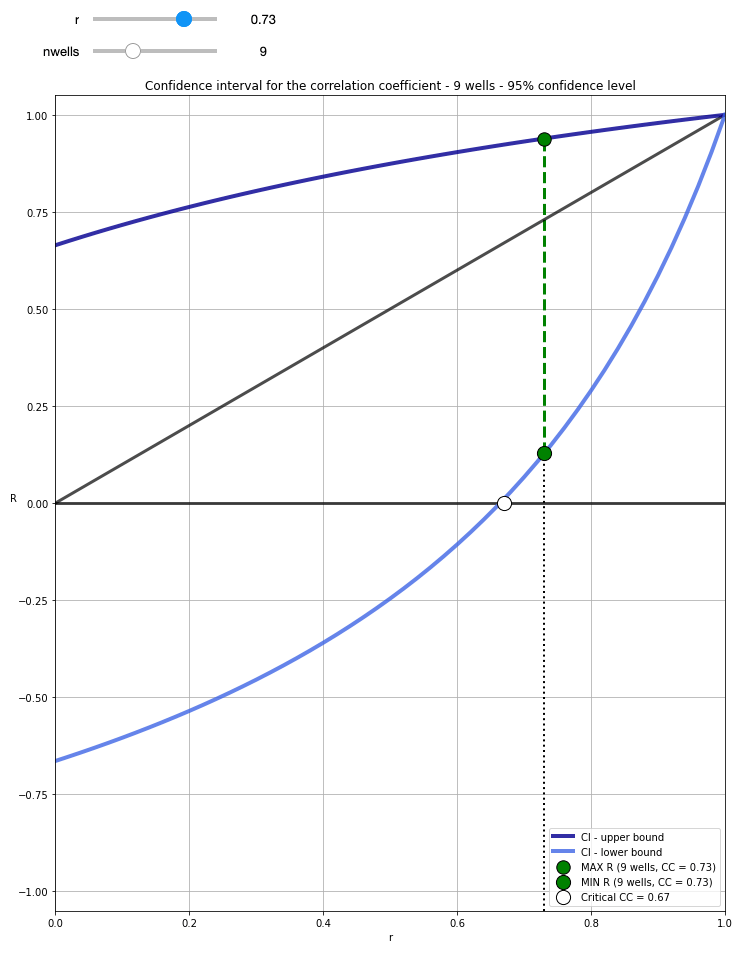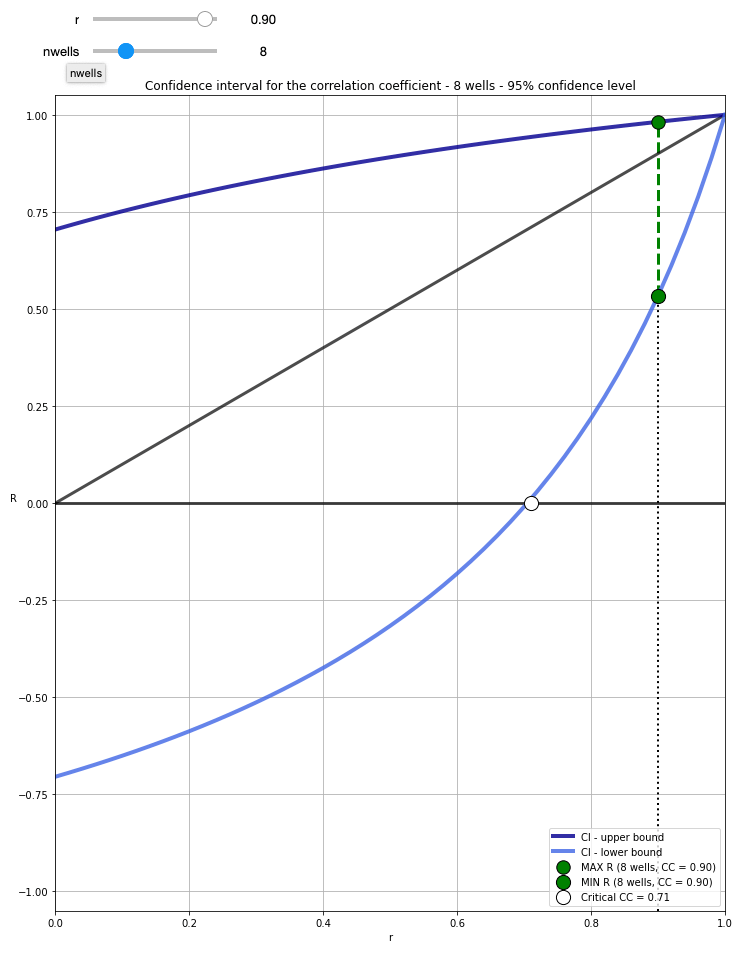With 9 wells, and cc=0.73, the resulting critical r = 0.67 tells us that for a 95% confidence level (0.05 alpha) we need at least a correlation coefficient of 0.67 in the sample (the 9 wells drilled) to be able to confidently say that there is correlation in the population (e.g. any well, future wells). However, the confidence interval is quite broad, ranging between 0.13 and 0.94 (you can get these numbers by running `confInt(0.73, 9)` in a cell.

With 8 wells (having removed the outlier), CC=0.9, the critical r is now 0.71, meaning that the requirement for rejecting the the null hypothesis (there is no association between Vp/Vs and Phi-H) is now a bit higher. However, a CC increased to 0.9, and only one less well, also results in a confidence interval ranging from 0.53 to 0.98, hence our confidence is greatly increased.

This second analysis also corroborates the choice of removing the outlier data point. One thing worth mentioning before moving on to the next test is that these confidence interval bounds are the expected population ones, based on the sample correlation coefficient and the number of observations; the data itself was not used. Of course, I could also have calculated, and shown you, the OLS regression confidence intervals, as I have done in this notebook (with a different dataset).

My final test involved using the distance correlation (`dcor.distance_correlation`) and p-value (`dcor.independence.distance_covariance_test`) from the `dcor` library. I have written before in a blog post how much I like the distance correlation, because it does not assume a linear relationship between variables, and because a distance correlation of zero does mean that there is no dependence between those two variables (contrary to Pearson and Spearman). In this case the relationship seems to be linear, but it is still a valuable test to compare DC and p-value before and after removing the outlier. Below is a summary of the test:
```All data points:
D.C. =  0.745
p-value =  0.03939
```
```Data without outlier:
D.C. =  0.917
p-value =  0.0012
```

The distance correlation values are very similar to the correlation coefficients from OLS, again going up once removed the outlier. But, more interestingly, with all data points, a p-value of 0.04079 is very close to the alpha of 0.05, whereas once we removed the outlier, the p-value goes down by a factor of 20, to 0.0018. This again backs up the decision to remove the outlier data point.

## From zero to JupyterLab pro on Windows 10

### Featured

N.B. last tested successfully onSepotember 27th, 2022.

I love JupyterLab, I really do! In my experience to date it proved to be the best environment for prototyping scientific computing applications interactively using Jupyter notebooks.

If you wonder if this is the right tool for you, please browse the rich documentation on the JupyterLab Interface and on how to work with Notebooks, then make sure to watch the 2018 Scipy tutorial. I guarantee that if you’ve been working with Jupyter notebooks and liked them, you will easily switch to JupyterLab and will never look back, it is only natural (also check Terraforming Jupyter to get a flavor of how much you can customize this environment to suit your needs).

Three times in the last couple of months I’ve had to make an installation from scratch on Windows 10 operated computers, using the Anaconda Python distribution: for a coworker’s desktop computer and my own, and for a friend on a laptop.

I’ve decided to summarize in this post my installation, which includes setting up JupyterLab and also creating virtual environments. I am hoping that even an absolute beginner will be able to follow these instructions, and go from zero to JupyterLab pro.

### Setting up JupyterLab with virtual environments on Windows 10

Go to the Ananaconda website for the Windows distribution and download the Python 3.8 installer: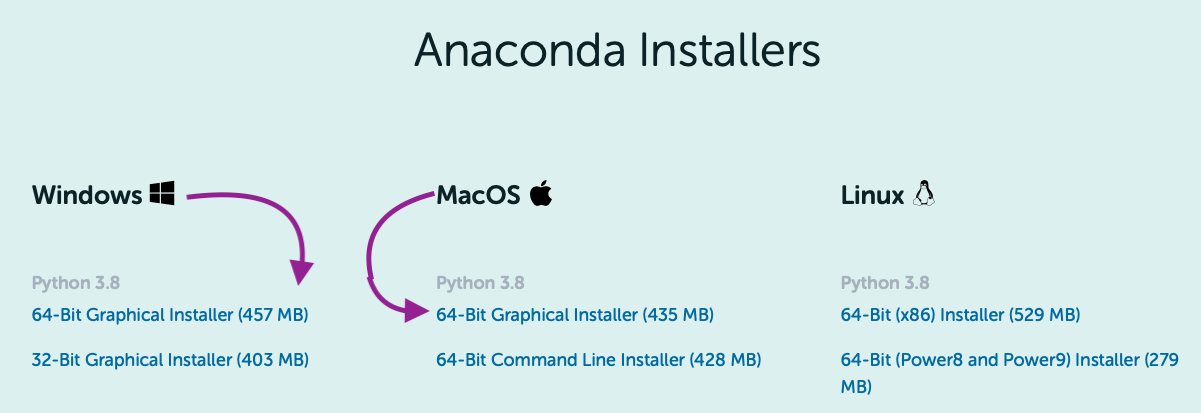NB: If you are one of those few still working with Python 2.7 (I was one until last fall), worry not, I will show you how to create a Python 2.7 virtual environment without much effort.

#### Step 2 – Install Anaconda

Follow the official installation instructions to the letter, with the exception of step 8. Here I would suggest especially if you want the ability to start JupyterLab from the command prompt, the alternative setting:NB: I realize this is discouraged because it may cause interference with other software down the road, but I’ve found no issue yet (not a guarantee, of course, so do at your own peril), and it is much easier than having to add the path manually.

#### Step 3 – Set Chrome as web browser for JupyterLab

I’ve never been able to make JupyterLab work with Internet Explorer, so this is not an optional step for me. To set Chrome as the browser for JupyterLab, open the config file `jupyter_notebook_config.py` (located in your home directory, in `~/.jupyter`), find the browser section:then replace the last line, `c.NotebookApp.browser = ''` , with:

`c.NotebookApp.browser = '"C:/path/to/your/chrome.exe" %s'` for example:

`‘”C:\Program Files\Google\Chrome\Application\chrome.exe” %s’`

N.B. For mac users, use this:

``c.NotebookApp.browser = 'open -a /Applications/Google\ Chrome.app %s'``

If the config file is not present in the home directory, it can be created at the prompt with the command:

``jupyter notebook --generate-config``

In Windows, this will create the file at this location:

`C:\Users\yourusername\.jupyter\jupyter_notebook_config.py`

#### Step 4 – Install nb_condas_kernels

The package nb_conda_kernels will later detect all conda environments that have notebook kernels and automatically registers them. As a result, all those environments will be visible and can be used directly from the JupyterLab interface. So:

a) check if `nb_conda_kernels` is installed by executing `conda list` at the prompt.

b) If you do not see it in the list of packages, then execute `conda install -c anaconda nb_conda_kernels`

#### Step 5 – Edit the Conda configuration file to create environments with default packages

To automatically install specific packages every time a new environment is created, add the package list to the create_default_packages section of the`.condarc` configuration file, which is located in the home directory. For example, in Windows, this would be:

`C:\Users\yourusername\.condarc`

If the `.condarc` configuration file is not present, you can:

• generate one using`conda config`, for example with:
``conda config --add channels conda-forge``

then edit it. The syntax for the file is the same used in environment files; here’s an example:NB:  `ipykernel` is necessary if `nb_conda_kernels` is to detect the environments.

If you’d prefer to install each package individually, you can install `ipykernel` with:

`conda install ipykernel`

#### Step 6 – Create the desired environments

To create both Python 3.6 and a Python 2.7 environments, for example, execute at the prompt the two commands below:

` conda create -n py37 python=3.7`

`conda create -n py27 python=2.7`

#### Step 7 – Start JupyterLab

At the prompt, execute the command:

`Jupyter lab`

This will open the JupyterLab Interface automatically in Chrome. From there you can select File>New>Notebook and you will be prompted to select a Kernel as below, where you see that both environments just created are available:#### Step 8 – Have fun

You are all set to work with the Notebooks in JupyterLab.

PS: To ensure the packages defined in the Conda configuration file were included in both environments, I run this example from the Seaborn tutorial: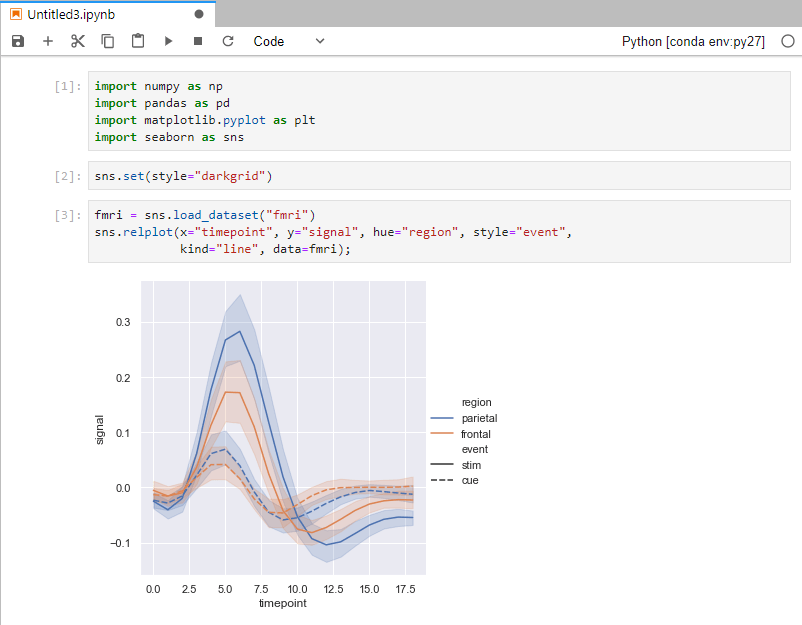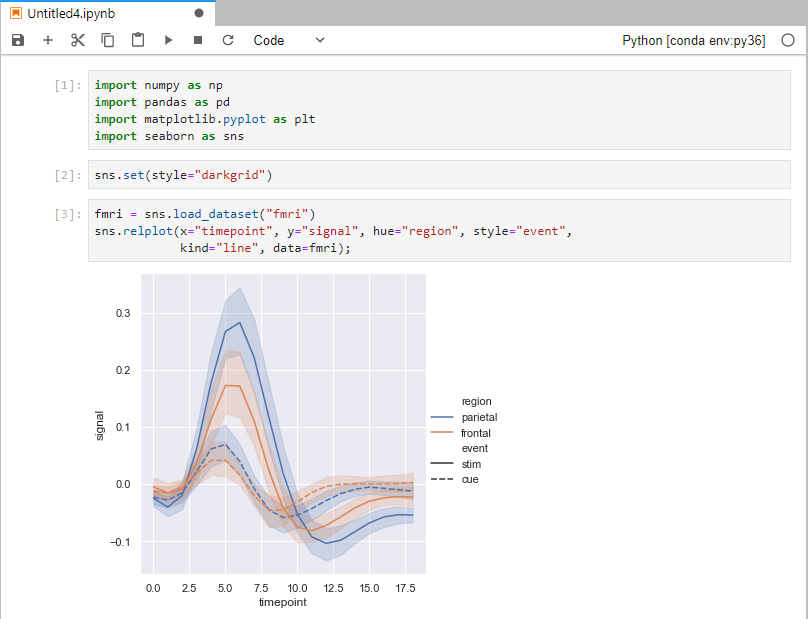## Data exploration in Python: distance correlation and variable clustering

### Featured

April 10, 2019

In my last post I wrote about visual data exploration with a focus on correlation, confidence, and spuriousness. As a reminder to aficionados, but mostly for new readers’ benefit: I am using a very small toy dataset (only 21 observations) from the paper Many correlation coefficients, null hypotheses, and high value (Hunt, 2013).

The dependent/target variable is oil production (measured in tens of barrels of oil per day) from a marine barrier sand. The independent variables are: Gross pay, in meters; Phi-h, porosity multiplied by thickness, with a 3% porosity cut-off; Position within the reservoir (a ranked variable, with 1.0 representing the uppermost geological facies, 2.0 the middle one, 3.0 the lowest one); Pressure draw-down in MPa. Three additional ‘special’ variables are: Random 1 and Random 2, which are range bound and random, and were included in the paper, and Gross pay transform, which I created specifically for this exercise to be highly correlated to Gross pay, by passing Gross pay to a logarithmic function, and then adding a bit of normally distributed random noise.

##### Correlation matrix with ellipses

I am very pleased with having been able to put together, by the end of it, a good looking scatter matrix that incorporated:

• bivariate scatter-plots in the upper triangle, annotated with rank correlation coefficient, confidence interval, and probability of spurious correlation
• contours in the lower triangle
• shape of the bivariate distributions (KDE) on the diagonal

In a comment to the post, Matt Hall got me thinking about other ways to visualize the correlation coefficient.  I did not end up using a colourmap for the facecolour of the plot (although this would probably be relatively easy, in an earlier attempt using hex-bin plots, the colourmap scaling of each plot independently – to account for outliers – proved challenging). But after some digging I found the Biokit library, which comes with a lot of useful visualizations, among which `corrplot` is exactly what I was looking for. With only a bit of tinkering I was able to produce, shown in Figure 1, a correlation matrix with:

• correlation coefficient in upper triangle (colour and intensity indicate whether positive or negative correlation, and its strength, respectively)
• bivariate ellipses in the lower triangle (ellipse direction and colour indicates whether positive or negative correlation; ellipticity and colour intensity are proportional to the correlation coefficient)Figure 1. Correlation matrix using the Biokit library

Also notice that – quite conveniently – the correlation matrix of Figure 1 is reordered with strongly correlated variables adjacent to one another, which facilitates interpretation. This is done using the rank correlation coefficient, with `pandas.DataFrame.corr`, and Biokit’s `corrplot`:

```corr = data.corr(method='spearman')
c = corrplot.Corrplot(corr)
c.plot(method='ellipse', cmap='PRGn_r', shrink=1, rotation=45, upper='text', lower='ellipse')
fig = plt.gcf()
fig.set_size_inches(10, 8);```

The insightful take-away is that with this reordering, the more ‘interesting’ variables, because of strong correlation (as defined in this case by the rank correlation coefficient), are close together and reposition along the diagonal, so we can immediately appreciate that Production, Phi-h, and Gross Pay, plus to a lesser extent position (albeit this one with negative correlation to production) are related to one another. This is a great intuition, and supports up our hypothesis (in an inferential test), backed by physical expectation, that production should be related to those other quantities.

But I think it is time to move away from either Pearson or Spearman correlation coefficient.

##### Correlation matrix with distance correlation and its p-value

I learned about distance correlation from Thomas when we were starting to work on our 2018 CSEG/CASP Geoconvention talk Data science tools for petroleum exploration and production“. What I immediately liked about distance correlation is that it does not assume a linear relationship between variables, and even more importantly, whereas with Pearson and Spearman a correlation value of zero does not prove independence between any two variables, a distance correlation of zero does mean that there is no dependence between those two variables.

Working on the R side, Thomas used the Energy inferential statistic package. With the`dcor` function he calculated the distance correlation, and with the `dcov.test `function the p-value via bootstrapping.

For Python, I used the `dcor` and `dcor.independence.distance_covariance_test` from the dcor library (with many thanks to Carlos Ramos Carreño, author of the Python library, who was kind enough to point me to the table of energy-dcor equivalents). So, for example, for one variable pair, we can do this:

```print ("distance correlation = {:.2f}".format(dcor.distance_correlation(data['Production'],
data['Gross pay'])))
print("p-value = {:.7f}".format(dcor.independence.distance_covariance_test(data['Production'],
data['Gross pay'],
exponent=1.0,
num_resamples=2000)))
>>> distance correlation  = 0.91
>>> p-value = 0.0004998```

So, wanting to apply these tests in a pairwise fashion to all variables, I modified the `dist_corr` function and `corrfunc` function from the existing notebook

```def dist_corr(X, Y, pval=True, nruns=2000):
""" Distance correlation with p-value from bootstrapping
"""
dc = dcor.distance_correlation(X, Y)
pv = dcor.independence.distance_covariance_test(X, Y, exponent=1.0, num_resamples=nruns)
if pval:
return (dc, pv)
else:
return dc```
```def corrfunc(x, y, **kws):
d, p = dist_corr(x,y)
#print("{:.4f}".format(d), "{:.4f}".format(p))
if p > 0.1:
pclr = 'Darkgray'
else:
pclr= 'Darkblue'
ax = plt.gca()
ax.annotate("DC = {:.2f}".format(d), xy=(.1, 0.99), xycoords=ax.transAxes, color = pclr, fontsize = 14)```

so that I can then just do:

```g = sns.PairGrid(data, diag_sharey=False)
axes = g.axes
g.map_upper(plt.scatter, linewidths=1, edgecolor="w", s=90, alpha = 0.5)
g.map_upper(corrfunc)
g.map_diag(sns.kdeplot, lw = 4, legend=False)
g.map_lower(sns.kdeplot, cmap="Blues_d")
plt.show();```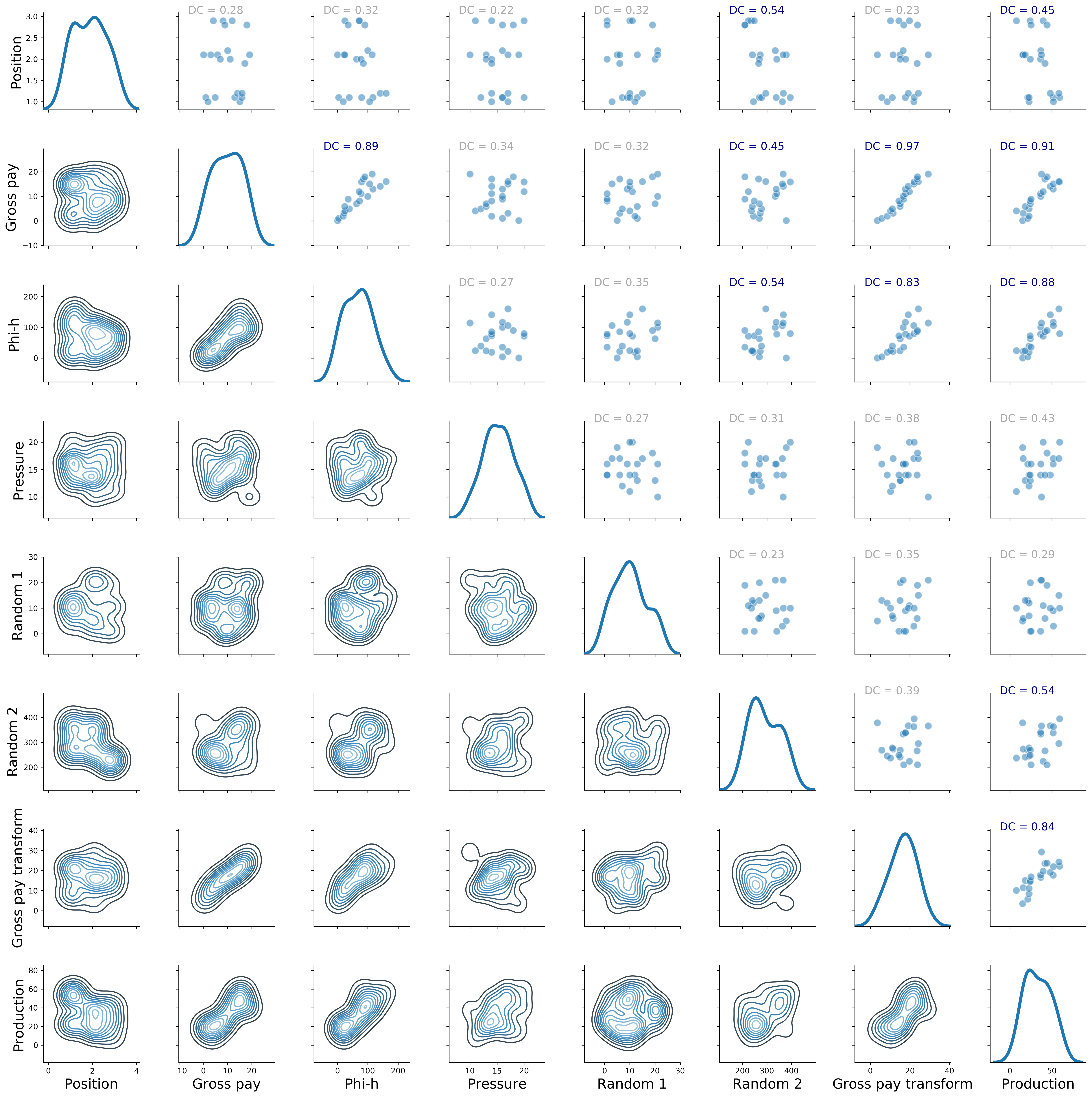Figure 2. Revised Seaborn pairgrid matrix with distance correlation colored by p-value (gray if p > 0.10, blue if p <= 0.10)

##### Clustering using distance correlation

I really like the result in Figure 2.  However, I want to have more control on how the pairwise plots are arranged; a bit like in Figure 1, but using my metric of choice, which would be again the distance correlation. To do that, I will first show how to create a square matrix of distance correlation values, then I will look at clustering of the variables; but rather than passing the raw data to the algorithm, I will pass the distance correlation matrix. Get ready for a ride!

For the first part, making the square matrix of distance correlation values, I adapted the code from this brilliant SO answer on Euclidean distance (I recommend you read the whole answer):

```# Create the distance method using distance_correlation
distcorr = lambda column1, column2: dcor.distance_correlation(column1, column2)
# Apply the distance method pairwise to every column
rslt = data.apply(lambda col1: data.apply(lambda col2: distcorr(col1, col2)))
# check output
pd.options.display.float_format = '{:,.2f}'.format
rslt
```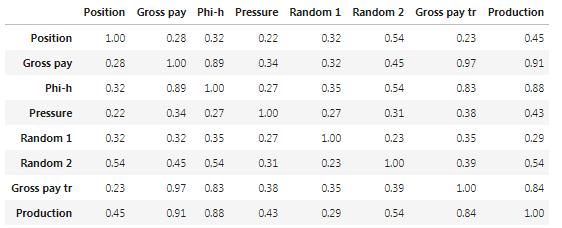Table I. Distance correlation matrix.

The matrix in Table I looks like what I wanted, but let’s calculate a couple of values directly, to be sure:

```print ("distance correlation = {:.2f}".format(dcor.distance_correlation(data['Production'],
data['Phi-h'])))
print ("distance correlation = {:.2f}".format(dcor.distance_correlation(data['Production'],
data['Position'])))```
```>>> distance correlation = 0.88
>>> distance correlation = 0.45```

Excellent, it checks!

Now I am going to take a bit of a detour, and use that matrix, rather than the raw data, to cluster the variables, and then display the result with a heat-map and accompanying dendrograms. That can be done with Biokit’s `heatmap`:

```data.rename(index=str, columns={"Gross pay transform": "Gross pay tr"}, inplace=True)
distcorr = lambda column1, column2: dcor.distance_correlation(column1, column2)
rslt = data.apply(lambda col1: data.apply(lambda col2: distcorr(col1, col2)))
h = heatmap.Heatmap(rslt)
h.plot(vmin=0.0, vmax=1.1, cmap='cubehelix')
fig = plt.gcf()
fig.set_size_inches(22, 18)
plt.gcf().get_axes().invert_xaxis();
```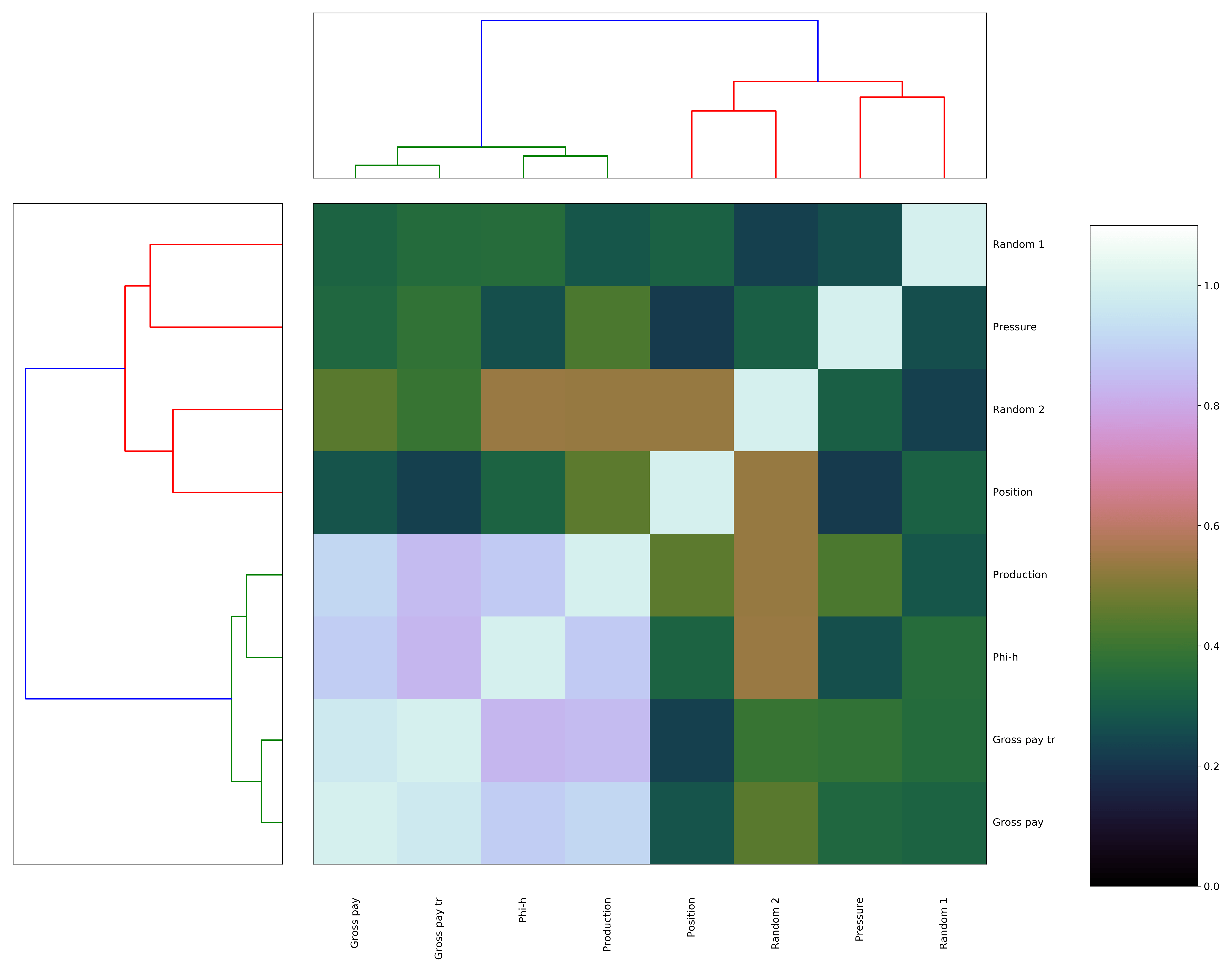Figure 3. Biokit heatmap with dendrograms, using correlation distance matrix

That’s very nice, but please notice how much ‘massaging’ it took: first, I had to flip the axis for the dendrogram for the rows (on the left) because it would be incorrectly reversed by default; and then, I had to shorten the name of Gross-pay transform so that its row label would not end up under the colorbar (also, the diagonal is flipped upside down, and I could not reverse it or else the colum labels would go under the top dendrogram). I suppose the latter too could be done on the Matplotlib side, but why bother when we can get it all done much more easily with Seaborn? Plus, you will see below that I actually have to… but I’m putting the cart before the horses…. here’s the Seaborn code:

```g = sns.clustermap(rslt, cmap="mako",  standard_scale =1)
fig = plt.gcf()
fig.set_size_inches(18, 18);```

and the result in Figure 4. We really get everything for free!Figure 4. Seaborn clustermap, using correlation distance matrix

Before moving to the final fireworks, a bit of interpretation: again what comes up is that Production, Phi-h, Gross Pay, and Gross pay transform group together, as in Figure 1, but now the observation is based on a much more robust metric. Position is not as ‘close’, it is ends up in a different cluster, although its distance correlation from Production is 0.45, and the p-value is <0.10, hence it is still a relevant variable.

I think this is as far as I would go with interpretation. It does also show me that Gross pay, and Gross pay transform are very much related to one another, with high dependence, but it does still not tell me, in the context of variable selection for predicting Production, which one I should drop: I only know it should be Gross pay transform because I created it myself. For proper variable selection I will look at techniques like Least Absolute Shrinkage and Selection Operator (LASSO, which Thomas has showcased in his R notebook) and Recursive Feature Elimination (I’ll be testing Sebastan Raschka‘s Sequential Feature Selector from the `mlxtend` library).

##### Correlation matrix with distance correlation, p-value, and plots rearranged by clustering

I started this whole dash by saying I wanted to control how the pairwise plots were arranged in the scatter matrix, and that to do so required use of  Seaborn. Indeed, it turns out the reordered row/column indices (in our case they are the same) can be easily accessed:

```a = (g.dendrogram_col.reordered_ind)
print(a)
>>> [1, 6, 2, 7, 0, 5, 3, 4]```

and if this is the order of variables in the original DataFrame:

```b = list(data)
print (b)
>>> [Position', 'Gross pay', 'Phi-h', 'Pressure', 'Random 1', 'Random 2', 'Gross pay transform', 'Production']```

we can rearrange them with those reordered column indices:

```data = data[[b[i] for i in a]]
print(list(data))
>>> ['Gross pay', 'Gross pay transform', 'Phi-h', 'Production', 'Position', 'Random 2', 'Pressure', 'Random 1']```

after which we can simply re-run the code below!!!

```g = sns.PairGrid(data, diag_sharey=False)
axes = g.axes
g.map_upper(plt.scatter, linewidths=1, edgecolor="w", s=90, alpha = 0.5)
g.map_upper(corrfunc)
g.map_diag(sns.kdeplot, lw = 4, legend=False)
g.map_lower(sns.kdeplot, cmap="Blues_d")
plt.show()
```Figure 5. Revised Seaborn pairgrid matrix with distance correlation colored by p-value (gray if p > 0.10, blue if p <= 0.10), and plots rearranged by clustering results

# Geoscience Machine Learning bits and bobs – data completeness

#### 2016 Machine learning contest – Society of Exploration Geophysicists

In a previous post I showed how to use `pandas.isnull` to find out, for each well individually, if a column has any null values, and `sum` to get how many, for each column. Here is one of the examples (with more modern, pandaish syntax compared to the example in the previous post:

```for well, data in training_data.groupby('Well Name'):
print(well)
print (data.isnull().values.any())
print (data.isnull().sum(), '\n')
```

Simple and quick, the output showed met that  – for example – the well ALEXANDER D is missing 466 samples from the PE log:

```ALEXANDER D
True
Facies         0
Formation      0
Well Name      0
Depth          0
GR             0
ILD_log10      0
DeltaPHI       0
PHIND          0
PE           466
NM_M           0
RELPOS         0
dtype: int64```

A more appealing and versatile alternative, which I discovered after the contest, comes with the `matrix` function form the missingno library. With the code below I can turn each well into a Pandas DataFrame on the fly, then a missingno matrix plot.

```for well, data in training_data.groupby('Well Name'):

msno.matrix(data, color=(0., 0., 0.45))
fig = plt.gcf()
fig.set_size_inches(20, np.round(len(data)/100)) # heigth of the plot for each well reflects well length
axes=fig.get_axes()
axes.set_title(well, color=(0., 0.8, 0.), fontsize=14, ha='center')```

I find that looking at these two plots provides a very compelling and informative way to inspect data completeness, and I am wondering if they couldn’t be used to guide the strategy to deal with missing data, together with domain knowledge from petrophysics.

Interpreting the dendrogram in a top-down fashion, as suggested in the library documentation, my first thoughts are that this may suggest trying to predict missing values in a sequential fashion rather than for all logs at once. For example, looking at the largest cluster on the left, and starting from top right, I am thinking of testing use of GR to first predict missing values in RDEP, then both to predict missing values in RMED, then DTC. Then add CALI and use all logs completed so far to predict RHOB, and so on.

Naturally, this strategy will need to be tested against alternative strategies using lithology prediction accuracy. I would do that in the context of learning curves: I am imagining comparing the training and crossvalidation error first using only non NaN rows, then replace all NANs with mean, then compare separately this sequential log completing strategy with an all-in one strategy.

# Busting bad colormaps with Python and Panel

I have not done much work with, or written here on the blog about colormaps and perception in quite some time.

Last spring, however, I decided to build a web-based app to show the effects of using a bad colormaps. This stemmed from two needs: first, to further my understanding of Panel, after working through the awesome tutorial by James Bednar, Panel: Dashboards (at PyData Austin 2019); and second, to enable people to explore interactively the effects of bad colormaps on their perception, and consequently the ability to on interpret faults on a 3D seismic horizon.

I introduced the app at the Transform 2020 virtual subsurface conference, organized by Software Underground last June. Please watch the recording of my lightning talk as it explains in detail the machinery behind it.

I am writing this post in part to discuss some changes to the app. Here’s how it looks right now: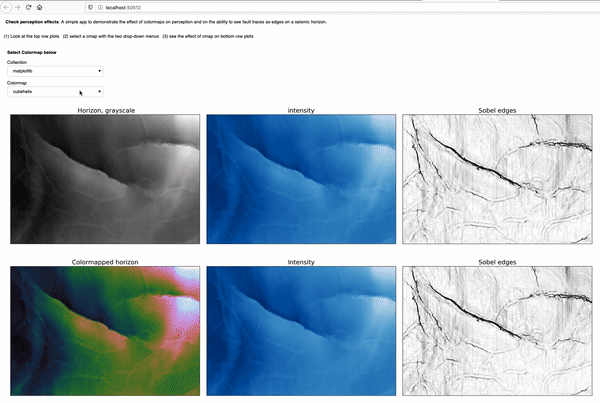The most notable change is the switch from one drop-down selector to two-drop-down selectors, in order to support both the Matplotlib collection and the Colorcet collection of colormaps. Additionally, the app has since been featured in the resource list on the Awesome Panel site, an achievement I am really proud of.You can try the app yourself by either running the notebook interactively with Binder, by clicking on the button below:```https://mybinder.org/v2/gh/mycarta/Colormap-distorsions-Panel-app/master?urlpath=%2Fpanel%2FDemonstrate_colormap_distortions_interactive_Panel
```

Let’s look at a couple of examples of insights I gained from using the app. For those that jumped straight to this example, the top row shows:

• the horizon, plotted using the benchmark grayscale colormap, on the left
• the horizon intensity, derived using `skimage.color.rgb2gray`, in the middle
• the Sobel edges detected on the intensity, on the right

and the bottom row,  shows:

• the horizon, plotted using the Matplotlib `gist_rainbow` colormap, on the left
• the intensity of the colormapped, in the middle. This is possible thanks to a function that makes a figure (but does not display it), plots the horizon with the specified colormap, then saves plot in the canvas to an RGB numpy array
• the Sobel edges detected on the colormapped intensity, on the rightI think the effects of this colormaps are already apparent when comparing the bottom left plot to the top left plot. However, simulating perception can be quite revealing for those that have not considered these effects before. The intensity in the bottom middle plot is very washed out in the areas corresponding to green color in the bottom left, and as a result, many of the faults are not visible any more, or only with much difficulty, which is demonstrated by the Sobel edges in the bottom right.

And if you are not quite convinced yet, I have created these hill-shaded maps, using Matt Hall”s delightful function from this notebook (and check his blog post):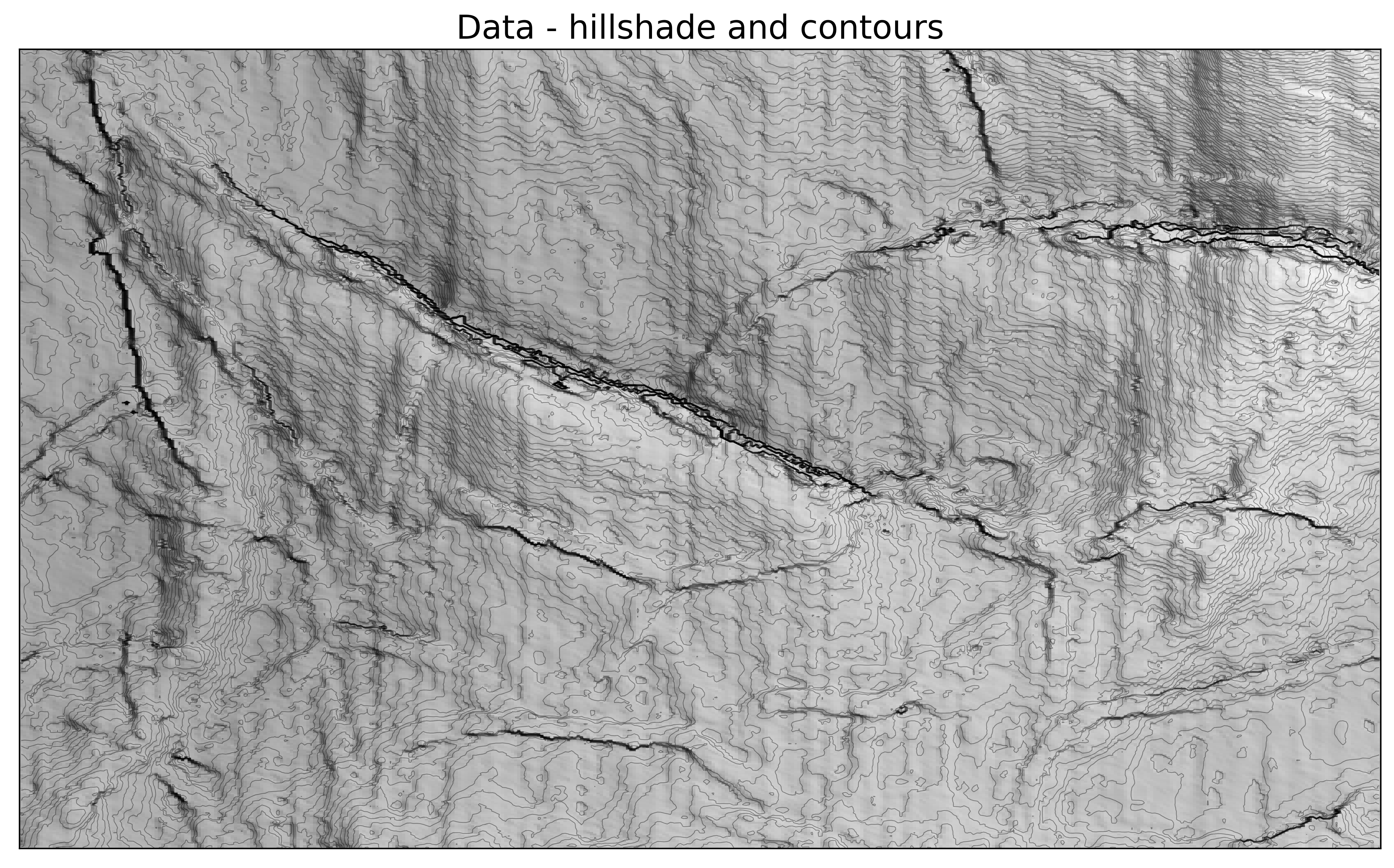Below is another example, using the Colocrcet `cet_rainbow` which is is one of Peter Kovesi’s perceptually uniform colormaps.  I use many of Peter’s colormaps, but never used this one, because I use my own perceptual rainbow, which does not have  a fully saturated yellow, or a fully saturated red. I think the app demonstrate, that even though they are more subtle , this rainbow still is introducing some artifacts. The yellow colour creates narrow flat bands, visible in the intensity and Sobel plots, and indicated by yellow arrows; the red colour is also bad as usual, causing an artificial decrease in intensity(magenta arrows).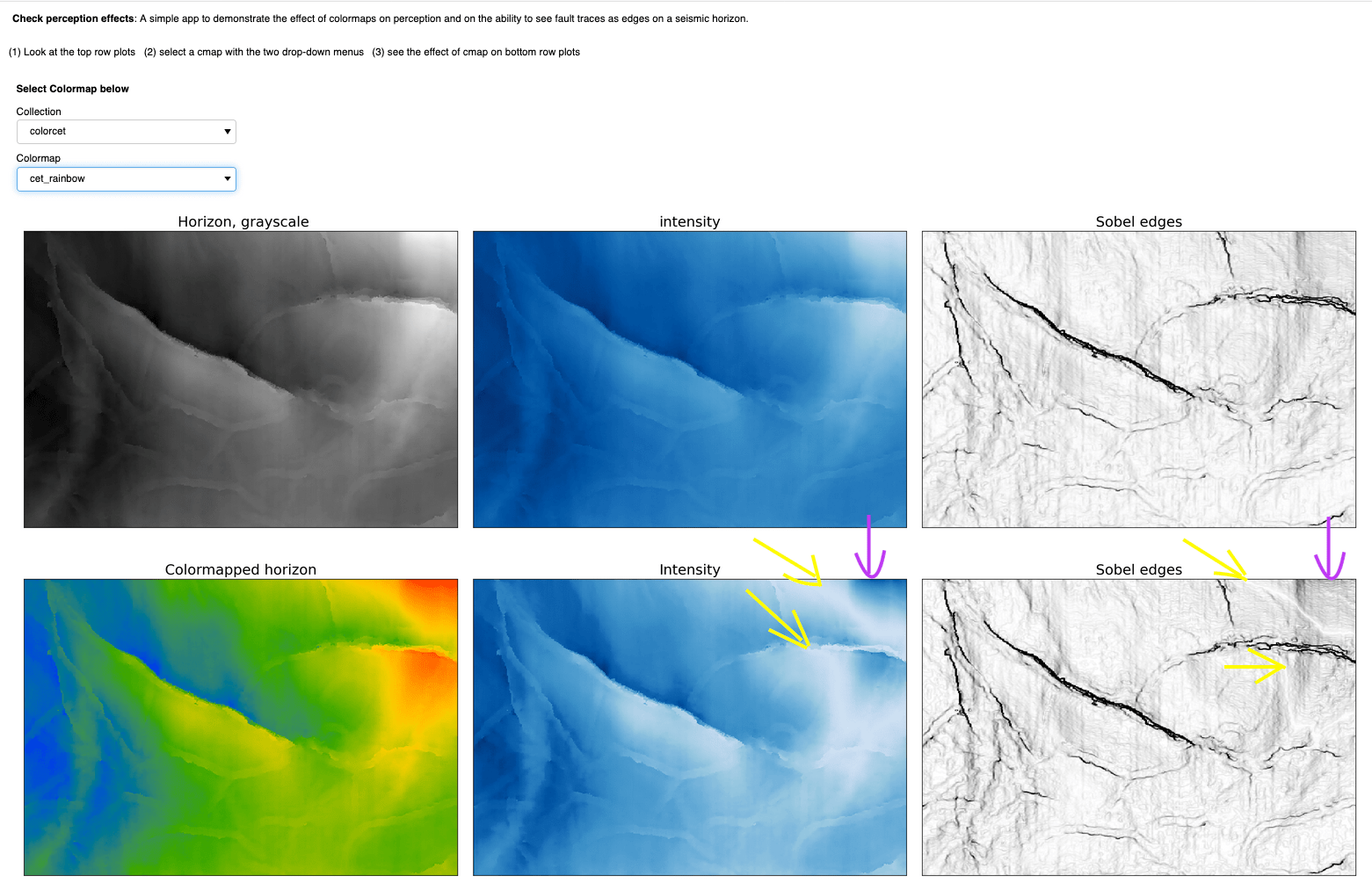# Upcoming book: 52 things you should know about Geocomputing

I am very excited to write that, after a successful second attempt at collecting enough essays, the book 52 Things You Should Know About Geocomputing, by Agile Libre, is very likely to become a reality.

#### *** July 2020 UPDATE ***

This project got a much needed boost during the hackathon at the 2020 Transform virtual event. Watch the video recording on the group YouTube channel here.

#### *********************

In one of the three chapter I submitted for this book, Prototype colourmaps for fault interpretation, I talk about building a widget for interactive generation of grayscale colourmaps with sigmoid lightness. The whole process is well described in the chapter and showcased in the accompanying GitHub repo.

This post is an opportunity for me to reflect on how revisiting old projects is very important. Indeed, I consider it an essential part of how I approach scientific computing, and a practical way to incorporate new insights, and changes (hopefully betterments) in your coding abilities.

In the fist version of the Jupyter notebook, submitted in 2017, all the calculations and all the plotting commands where packed inside a single monster function that was passed to `ipywidgets.interact`. Quite frankly, as time passed this approach seemed less and less Phytonic (aka mature) and no longer representative of my programming skills and style, and increased understanding of widget objects.

After a significant hiatus (2 years) I restructured the whole project in several ways:
– Converted Python 2 code to Python 3
– Created separate helper functions for each calculation and moved them to the top to improve on both clarity and reusability of the code.
– Improved and standardized function docstrings
– Optimized and reduced the number of parameters
– Switched from `interact` to `interactive` to enable access to the colormaparray in later cells (for printing, further plotting, and exporting).

The new notebook is here.

In a different chapter in the book I talk at more length about the need to Keep on improving your geocomputing projects.

.